1. /
2. CBSE
3. /
4. Class 08
5. /
6. Mathematics
7. /
8. NCERT Solutions for Class...

# NCERT Solutions for Class 8 Maths Exercise 9.2

NCERT solutions for Class 8 Maths Algebraic Expressions and Identities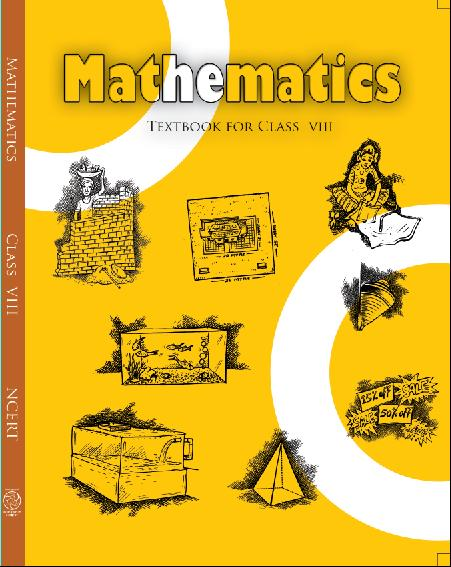## NCERT Solutions for Class 8 Maths Algebraic Expressions and Identities

###### 1. Find the product of the following pairs of monomials:

(i)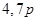(ii)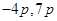(iii)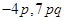(iv)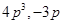(iv)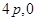Ans.

(i)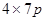=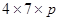=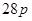(ii)=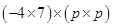=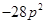(iii)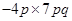=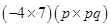=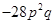(iv)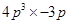=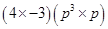=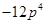(v)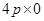=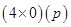= 0

NCERT Solutions for Class 8 Maths Exercise 9.2

###### 2. Find the areas of rectangles with the following pairs of monomials as their lengths and breadths respectively: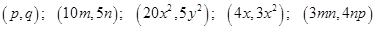Ans.

(i)   Area of rectangle

=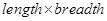=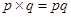sq. units

(ii) Area of rectangle

==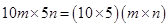=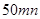sq. units

(iii) Area of rectangle  ==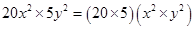=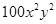sq. units

(iv) Area of rectangle  ==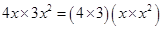=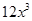sq. units

(v) Area of rectangle  ==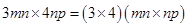=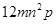sq. units

NCERT Solutions for Class 8 Maths Exercise 9.2

###### 3. Complete the table of products:

(i)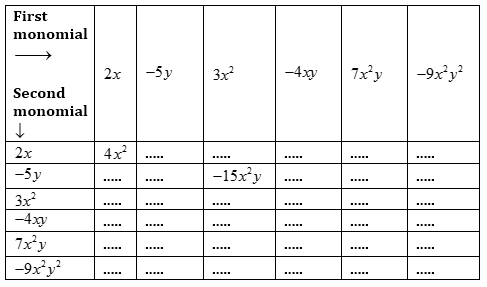Ans.

(i)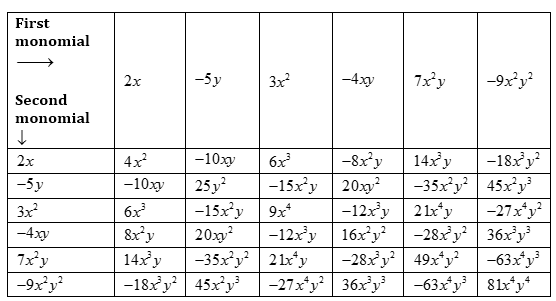NCERT Solutions for Class 8 Maths Exercise 9.2

###### 4. Obtain the volume of rectangular boxes with the following length, breadth and height respectively:

(i)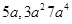(ii)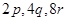(iii)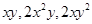(iv)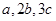Ans. (i)   Volume of rectangular box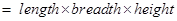=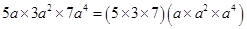=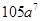cubic units

(ii) Volume of rectangular box=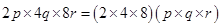=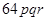cubic units

(iii) Volume of rectangular box=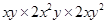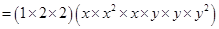=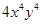cubic units

(iv) Volume of rectangular box=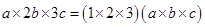=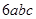cubic units

NCERT Solutions for Class 8 Maths Exercise 9.2

###### 5. Obtain the product of:

(i)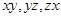(ii)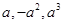(iii)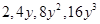(iv)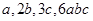(v)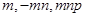Ans.

(i)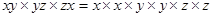=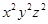(ii)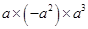=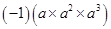=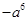(iii)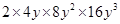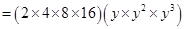=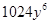(iv)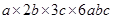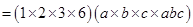=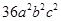(v)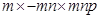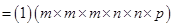=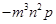## NCERT Solutions for Class 8 Maths Exercise 9.2

NCERT Solutions Class 8 Mathematics PDF (Download) Free from myCBSEguide app and myCBSEguide website. Ncert solution class 8 Mathematics includes text book solutions from Class 8 Maths Book . NCERT Solutions for CBSE Class 8 Maths have total 16 chapters. 8 Maths NCERT Solutions in PDF for free Download on our website. Ncert class 8 solutions PDF and Maths ncert class 8 PDF solutions with latest modifications and as per the latest CBSE syllabus are only available in myCBSEguide.

## CBSE app for Class 8

To download NCERT Solutions for class 8 Social Science, Computer Science, Home Science,Hindi ,English, Maths Science do check myCBSEguide app or website. myCBSEguide provides sample papers with solution, test papers for chapter-wise practice, NCERT solutions, NCERT Exemplar solutions, quick revision notes for ready reference, CBSE guess papers and CBSE important question papers. Sample Paper all are made available through the best app for CBSE students and myCBSEguide website.### Test Generator

Create question paper PDF and online tests with your own name & logo in minutes.### myCBSEguide

Question Bank, Mock Tests, Exam Papers, NCERT Solutions, Sample Papers, Notes

### 7 thoughts on “NCERT Solutions for Class 8 Maths Exercise 9.2”

1. It is a good app for students

2. Thanks it helped me for my annual exams very well. The main reasin that i used this webpage is that my answer page in my ncert maths text book had teared out and i was unable to make sure that my answer was right or not. This site helped me very well.Thankzz

3. v helpful site thank u so much

4. Thank????????
????????
????????
????????
????????

5. Thankful

6. Very helpful site for doing homeworks

7. it is a good app
I am a student